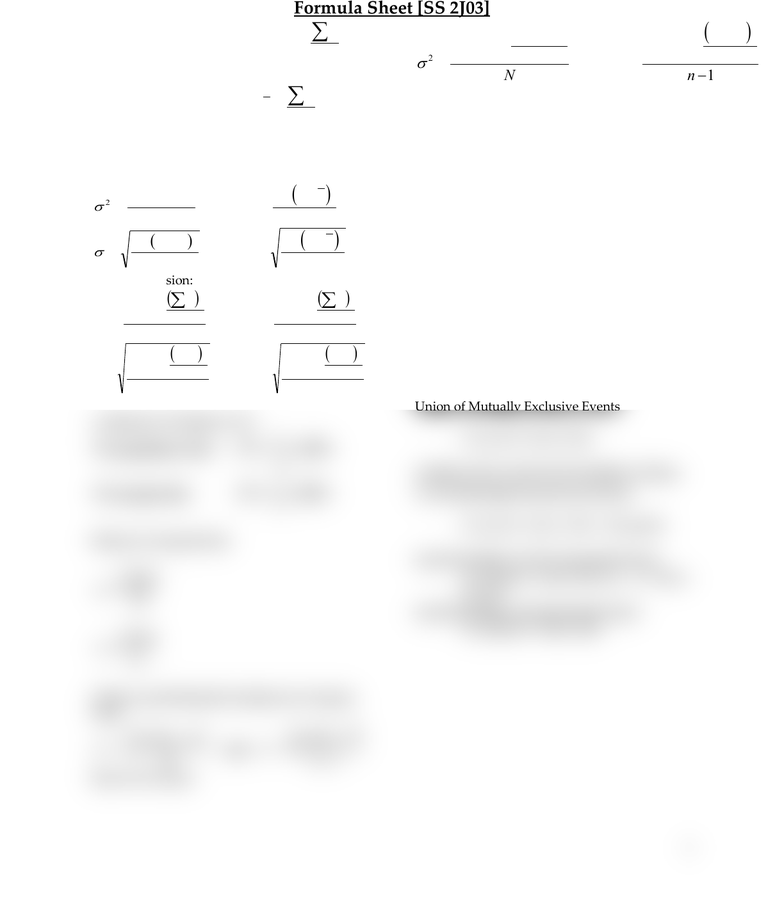# SOCSCI 2J03 Quiz: Stats Formula Sheet-Test-1

29 views1 pages1
Formula Sheet [SS 2J03]
Mean for population data:
N
x
=
Mean for sample data:
n
x
x
=
Variance and Standard Deviation for
Ungrouped Data
( )
( )
( )
( )
1
and
1
and
2
2
2
2
2
2
=
=
=
=
n
xx
s
N
x
n
xx
s
N
x
Short-cut version:
( ) ( )
( ) ( )
1
and
1
and
2
2
2
2
2
2
2
2
2
2
=
=
=
=
n
n
x
x
s
N
N
x
x
n
n
x
x
s
N
N
x
x
Coefficient of Variation (CV):
%100 CV :data sampleFor
%100 CV :data populationFor
=
=
x
s
Mean for Grouped Data:
N
mf
=
n
mf
x
=
Variance and Standard Deviation for Grouped
Data:
( )
( )
1
and
2
2
2
2
=
=
n
xmf
s
N
mf
Short-Cut version:
Chebyshev’s Theorem:
For any number k greater than 1, at least
(1 1/k²) of the data values lie within k
standard deviations of the mean.
Empirical Rule:
For a bell-shaped distribution, approximately
68% of the observations lie within one
standard deviation of the mean
95% of the observations lie within two
standard deviations of the mean
99.7% of the observations lie within
three standard deviations of the mean
Addition Rule to Find the Probability of the
Union of Mutually Exclusive Events
P (A or B) = P(A) + P(B)
Addition Rule to Find the Probability of Union
of Two Mutually Nonexclusive Events
P (A or B) = P(A) + P(B) P(A and B)
Joint Probability of Two Dependent Events:
P (A and B) = P(A) x P(B |A) or P(B) x
P(A |B)
Joint Probability of Independent Events:
P (A and B) = P(A) x P(B)
Unlock document

This preview shows half of the first page of the document.
Unlock all 1 pages and 3 million more documents.